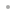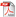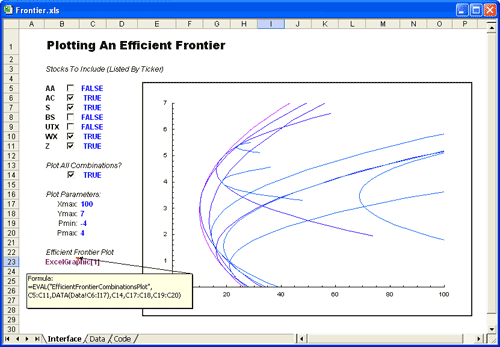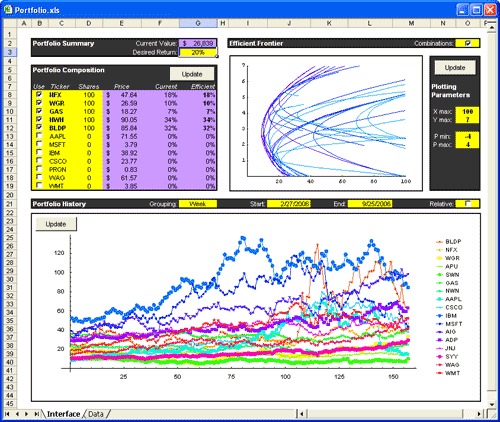MATHEMATICA LINK FOR EXCELMathematica Link for ExcelFeaturesWhat's New in Version 3Who's It For?ExamplesFinanceManagementEngineeringIndustryUser's Manual and Reference GuideBuy Online# Examples: Finance

Mathematica Link for Excel lets you easily process financial data and perform sophisticated analyses by using custom functions written in Mathematica. You can also use Mathematica-based macros to automatically calculate and visualize your data in Excel.

### Custom Mathematica Functions

In finance, the efficient frontier is the boundary of points that define the minimum variance achievable from a set of stocks for any given return. Here, we calculate and display an efficient frontier for a set of selected stocks. The information for these stocks is stored on the Data sheet in the workbook. Storing the functions apart from the data they operate on allows the investment logic to be more easily shared, reused and maintained.

Download example### Mathematica-Based Macros

Here, Mathematica calculates an optimal portfolio composition, visualizes the efficient frontier and displays the historical stock prices for the stocks selected. A Mathematica-based macro is stored in the Excel workbook, inside an Initialization code box. One click of the Mathematica Evaluate button runs the code and updates all Mathematica calculations.

Download example